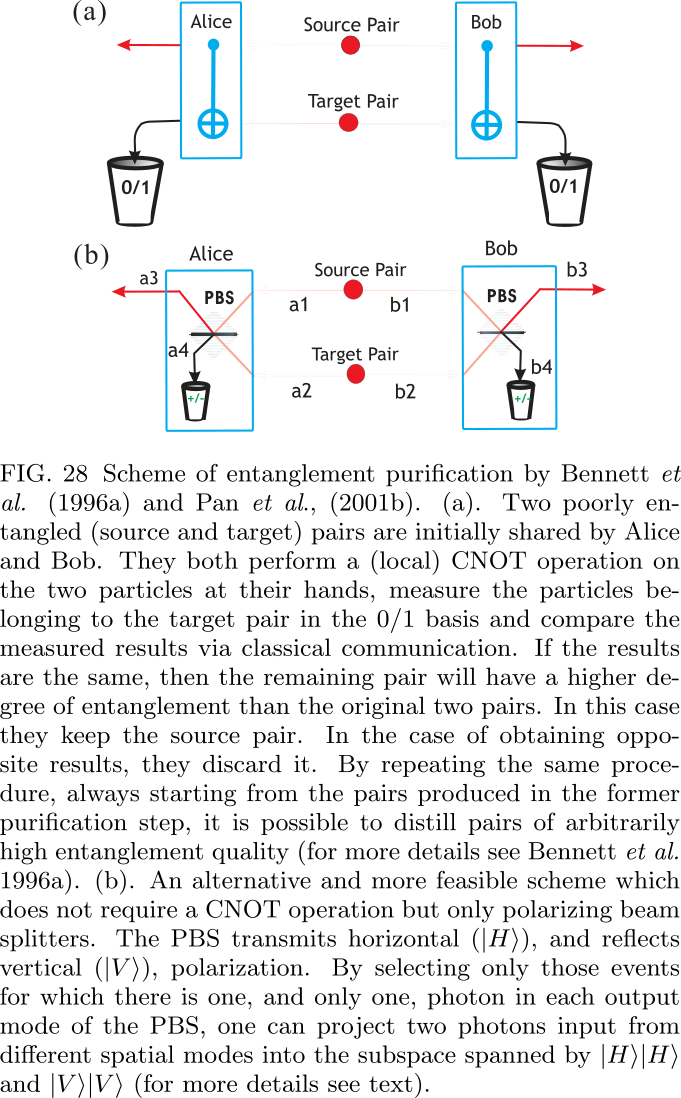# 1.4.2. Multi-photon entanglement and interferometry 

## 1.4.2.1. Abstract

Multi-photon interference reveals strictly non-classical phenomena. Its applications range from fundamental tests of quantum mechanics to photonic quantum information processing, where a significant fraction of key experiments achieved so far comes from multi-photon state manipulation. We review the progress, both theoretical and experimental, of this rapidly advancing research. The emphasis is given to the creation of photonic entanglement of various forms, tests of the completeness of quantum mechanics (in particular, violations of local realism), quantum information protocols for quantum communication (e.g., quantum teleportation, entanglement purification and quantum repeater), and quantum computation with linear optics. We shall limit the scope of our review to “few photon” phenomena involving measurements of discrete observables.

## 1.4.2.2. INTERFERENCE AND QUANTUM ENTANGLEMENT

We discuss here the basic differences between the classical interference understood as interference of electro-magnetic waves in space, and the quantum one which is interference of various operationally indistinguishable processes.

### 1.4.2.2.1. Classical interference

Consider two quasi-monochromatic plane waves linearly polarized in the same direction, described by finally c.c. denotes the complex conjugate of the previous expression. The intensity of the superposed fields at a certain point in space is given by The Hanbury Brown and Twiss experiment introduced intensity correlation measurements to optics. Such correlations between two points in space and two moments of time, for two classical fields are described by an intensity correlation function The average is taken over an ensemble, and for stationary fields this is equivalent to the temporal average. Even when no intensity variations are observable (i.e., for averaged intensity constant in space), the intensity correlations can reveal interference effects. Assume that the phases of the two fields fluctuate independently of one another. Then for , the function still ex-hibits a spatial modulation or maximal visibility of 50% as exhibited by the formula: where . This formula can be easily reached by noting that the temporal average of where , is given by and due to the random nature of only the first term survive Thus, the visibility of intensity correlations for fields with fluctuat- ing phase differences is never full, maximally 50%.

### 1.4.2.2.2. Quantum interference

#### Single-particle quantum interference

In the classical particle picture one would expect that a process originating with state A and with possible intermediate stages , leading to an event C, would be described by In the quantum case ,where The difference between (C) and (Q) is in the assumption, inherent in (C), that the particle had to be in one of the intermediate situations (states) . In the quantum case any attempt to verify by measurement 5 which of the situations actually took place puts one back to the classical formula (C). The formula (Q) leads to interference phenomena, and may be thought as a manifestation of a wave nature of quantum particles, whereas, if we make measurements discriminating events , we learn by which way (welcher weg) the particles travel. The “which-way information”is a clear signature of the particle nature.

#### Two-particle quantum interference

Consider such a correlation: assume that if particle 1 is at , then particle 2 is also at , and, whenever 1 is at then particle 2 is at . Later on the particles are detected at two different points, and . Then, according to the rules given above Thus, if the phase relation between the two amplitudes is stable one can have absolutely noiseless interference with 100% visibility, while there is no single particle interference: As we shall see, the unbounded visibility is not the only feature by which two-particle interference differs from classical one.(It seems that this paragraph is trying to compare the visibility between classical cases and quantum cases, but there may be some logic flaws, because it wants to compare the visibility of with that of probablity)

### 1.4.2.2.3. Quantum entanglement

where, e.g., describes the state of particle 1 with its spin up (down) along the z-direction. The minus sign is necessary to get the rotational invariance. The spin state of one of the particles is described by a reduced density operator 6 , which is a totally random state and is the unit operator for a given subsystem.

A hermitian operator is an entanglement witness if for all separable states one has , whereas there exists an entangled state for which one has . Thus, via measurement of a suitably chosen witness operator one can detect entanglement.

### 1.4.2.2.4. Interferometry with entangled two- and multi-photon states

#### EPR interferometry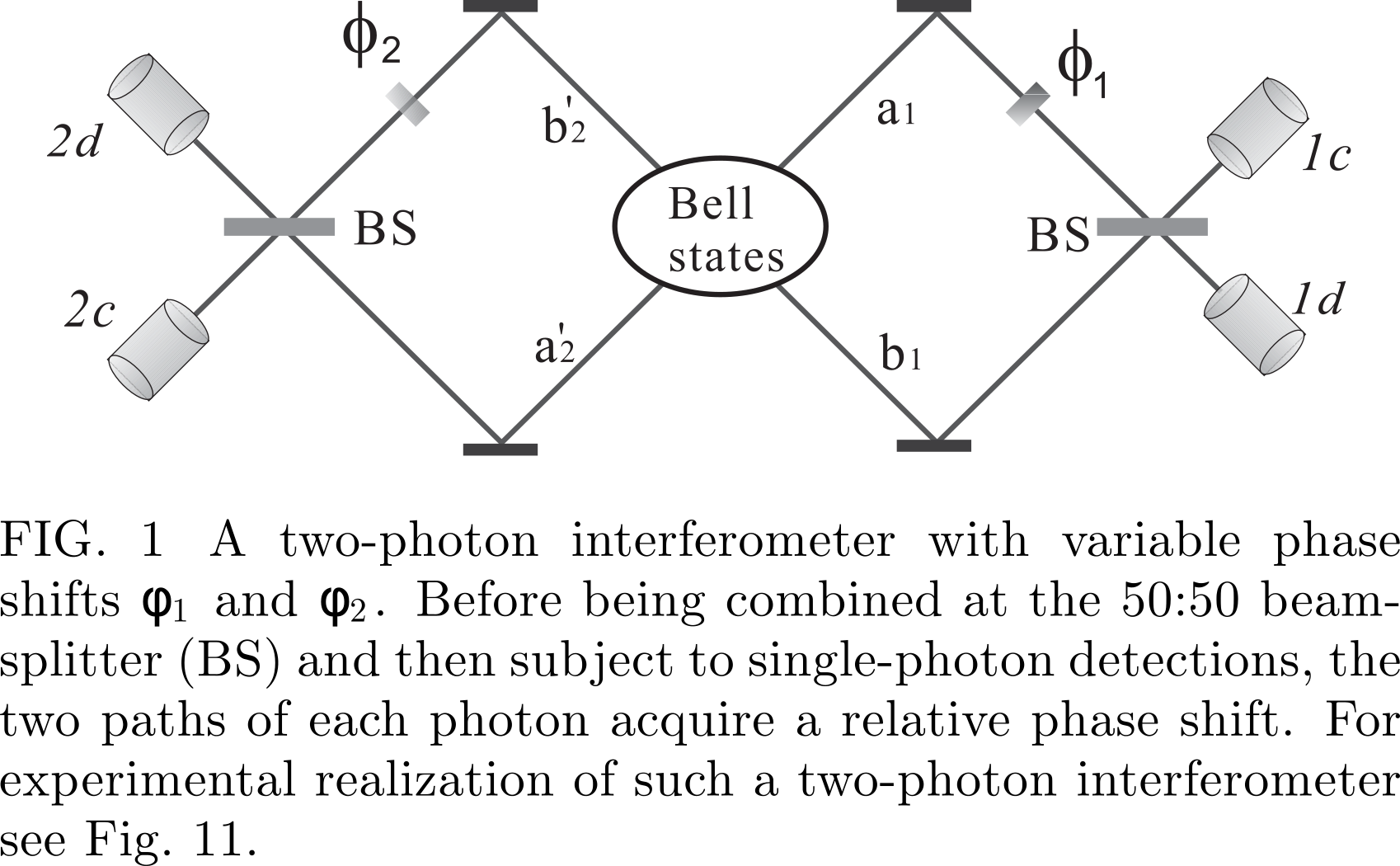We assume that a central source emits two photons in an entangled state By taking into account the phase shifts and (The terms times respectively) and the action of the two beamsplitters(,), the probabilities of the coincidence detections of two photons at the detector pairs ( ) read Thus, by simultaneously monitoring the detectors on both sides of the interferometer, while varying the phase shifts and , the interference fringes will be observed as shown by the sinusoidal terms. In contrast, for any single detector the count rate shows no interference at all.

#### GHZ interferometry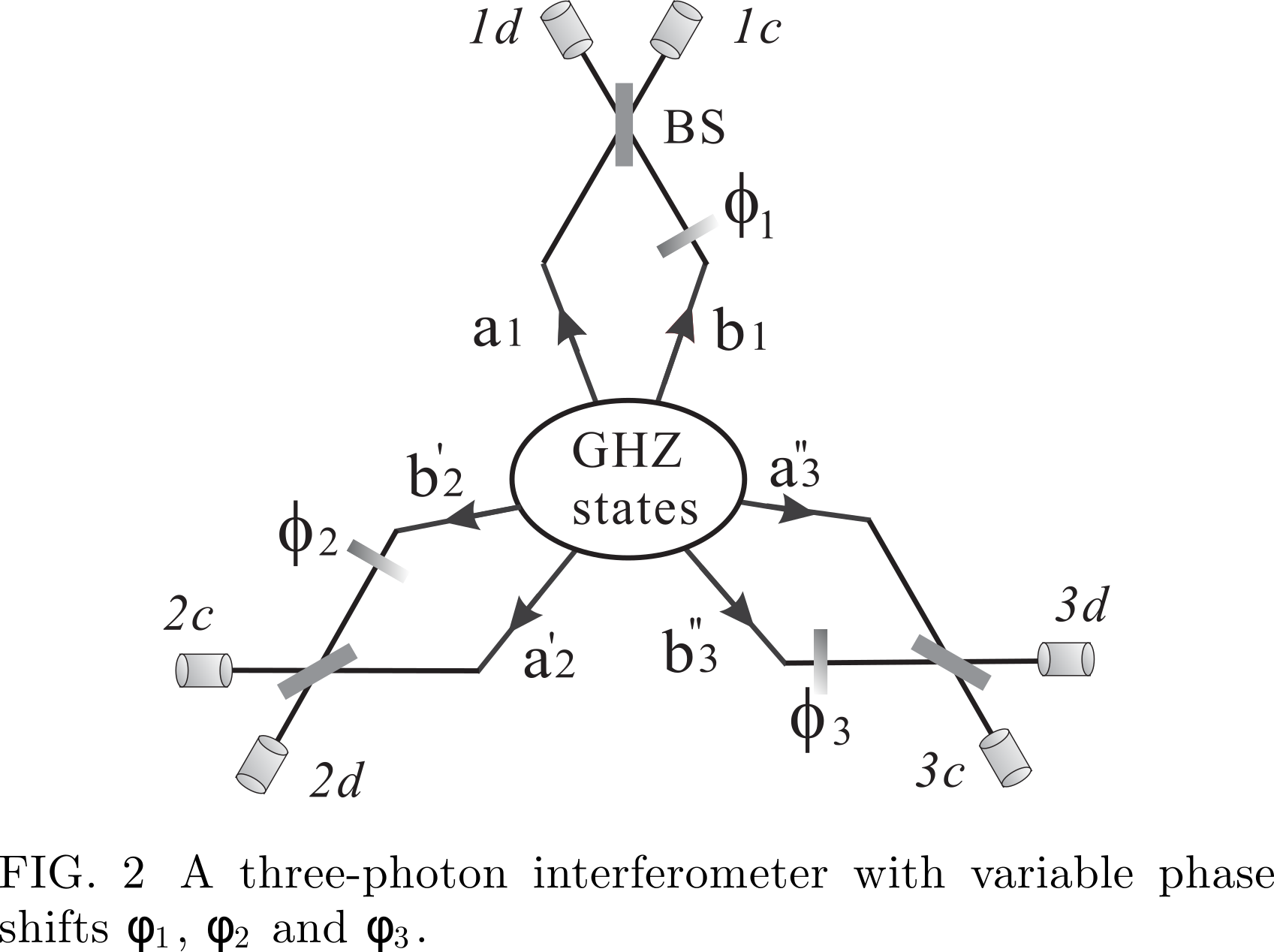## 1.4.2.3. PHOTONIC QUBITS AND LINEAR OPTICS

A general (normalized) basis state of the Fock space is of the following form: It is easy to see that if one defines the creation operators with respect to an alternative basis of wave packet profiles (here the primed ones) one has:

### 1.4.2.3.1. Photonic qubits

A quantum bit, or qubit, is the most elementary unit of quantum information. It is a generalization of the classical bit, which has two distinguishable states “0” and “1”. Similarly, we can have a qubit in two distinguishable, i.e., orthogonal states and . However, in contradistinction to its classical counterpart, a qubit can be prepared as, or transformed to, any superposition of these two states (normalization requires ): Any isolated two-level system consisting of a pair of orthogonal quantum states represents a qubit.

1. Polarization qubits they can easily be created and manipulated with high precision by simple linear-optical elements such as polarizing beam splitters (PBS), polarizers and wave plates.
2. Spatial qubits Any state of spatial qubits can be prepared by using suitable phase shifters and BSs. A disadvantage of using spatial qubits is that the coherence between and is sensitive to the relative phase between the paths a and b, and this is difficult to control in long-distance cases.
3. Time-bin qubits

Two-photon polarization entangled states. The so- called Bell states 14 form a basis in the four dimensional two-qubit Hilbert space. Bell states of photonic polarization qubits can be for example:

### 1.4.2.3.2. Simple linear-optical elements

In the photonic domain, quantum states of photons can be easily, and with a high precision, manipulated by simple passive linear-optical devices. These linear-optical elements include BS, polarizing beamsplitter (PBS), wave plates and phase shifters. Classically, such devices conserve energy: The total input energy equals the total output energy, and there is no energy transfer between different frequencies. A passive linear optical device is described by a unitary transformation of annihilation operators for the same frequency: where U is a unitary matrix, and the indices denote a basis of orthogonal modes. The BS is one of the most important optical elements.The most commonly used BS is the symmetric 50:50 BS characterized by the following transformation The factor i is a consequence of unitarity. It describes a phase jump upon reflection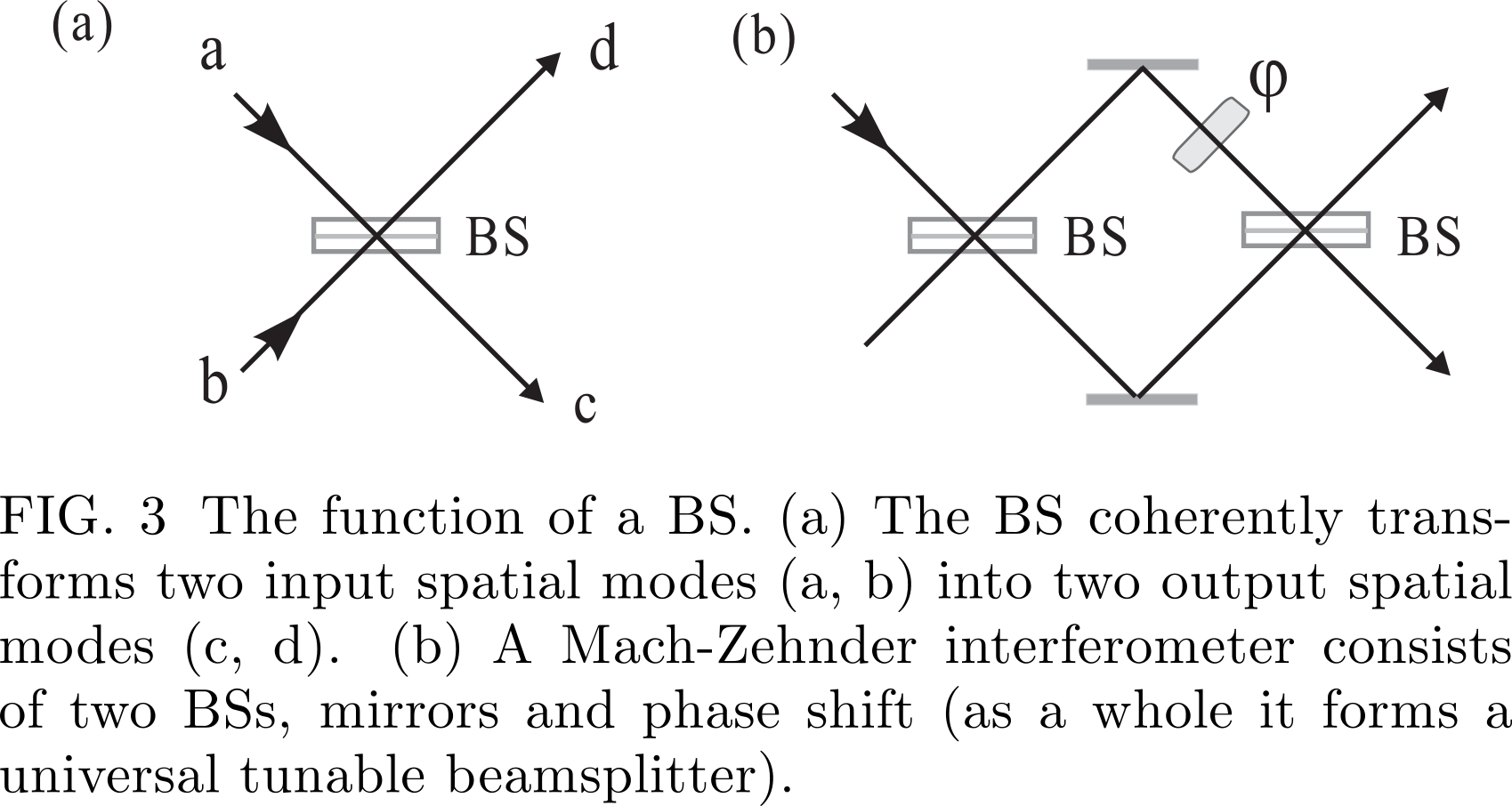Another important component is the polarizing beam-splitter (PBS)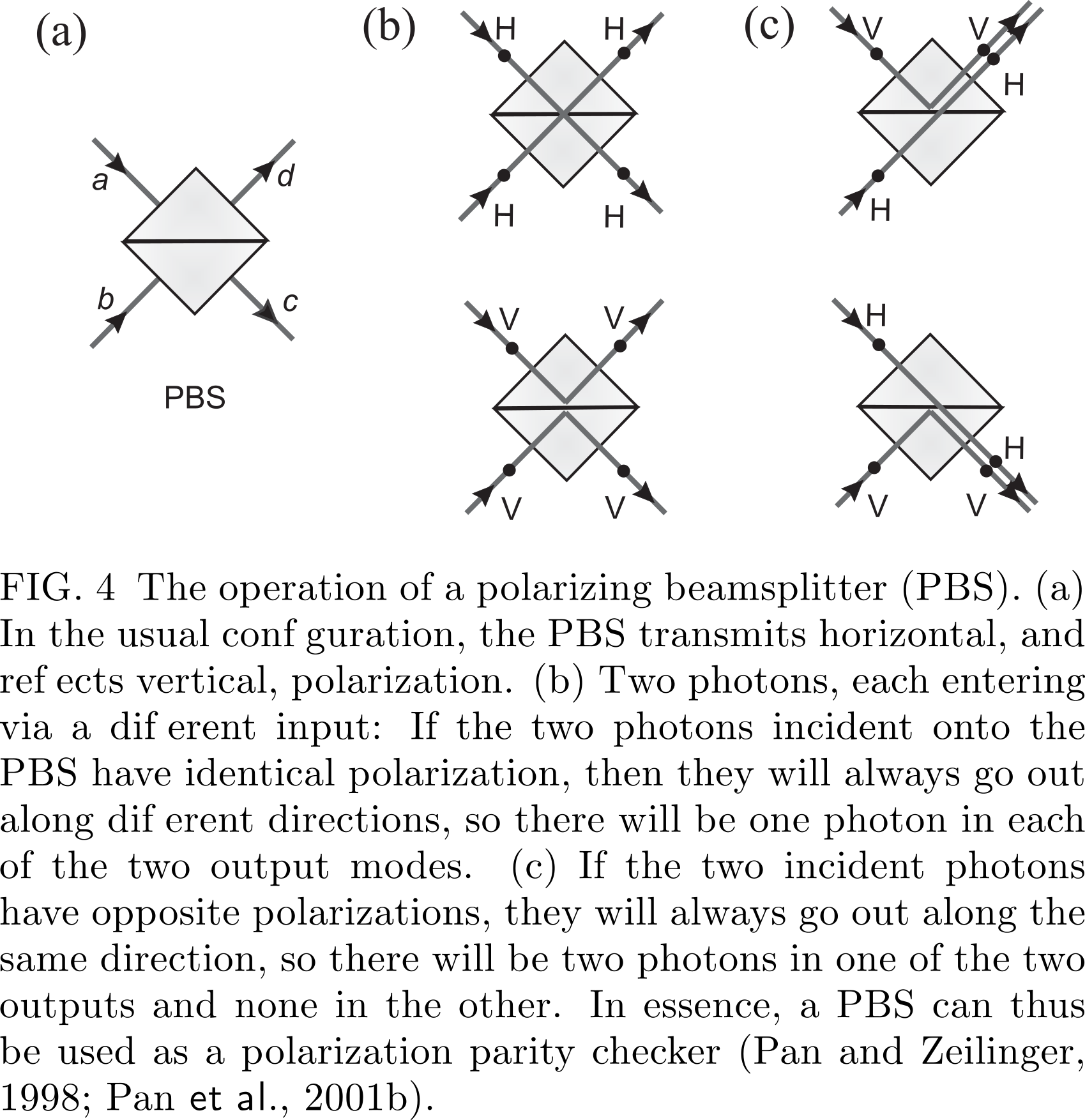### 1.4.2.3.3. Two-photon interference due to indistinguishability of photons

As we have seen previously, upon reflection off a symmetric 50:50 BS a photon picks a phase shift i. Denote the input modes as a and b and output modes as c and d. If we have two spectrally identical photons (of the same polarization) each entering at exactly the same moment an opposite input port of the BS, the initial state , is transformed into We have a cancellation of the two terms, which describe the cases in which each photon exits by a different exit port. This cancellation occurs if the two photons are perfectly indistinguishable in terms of all their other degrees of freedom such as frequency, time, or polarization.

If the photons are at least partially distinguishable (in this case we shall label the annihilation operators of the photons with different subscripts as and ) the initial state is transformed by a perfect 50:50 BS via the relations in Eqn.28 into

### 1.4.2.3.4. Post-selection of entanglement and quantum erasure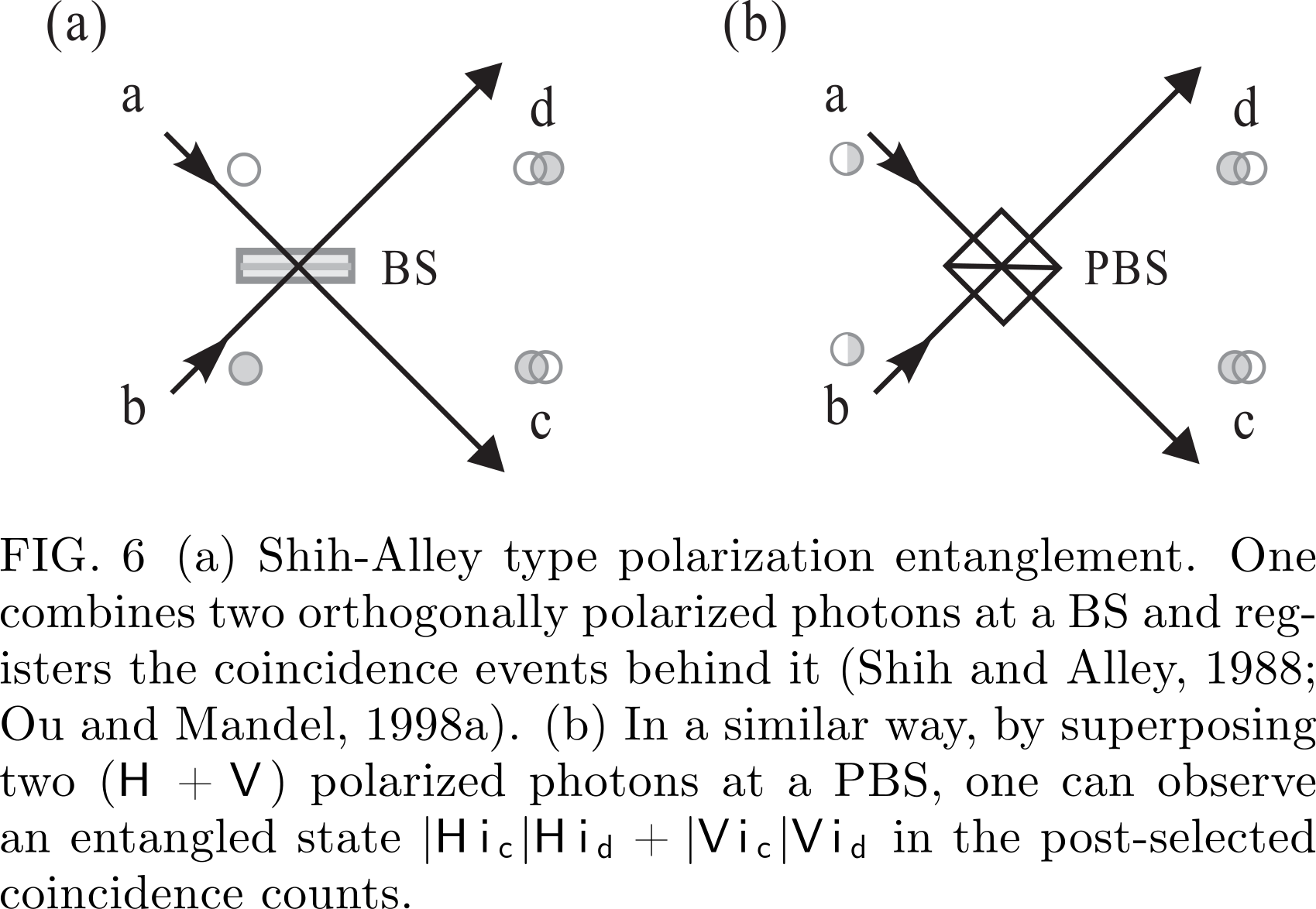When analyzing the above experiments, one could be mislead to suppose that the interference arises due to the fact that the wave packets of the two photons overlap at the BS. However, it is important to note that essentially, the origin of this interference is due to the indistinguishability of two-photon amplitudes describing the various alternatives leading to a coincidence count. To dispel a misconception that the photons must arrive at the BS at the same time for some type of classical local “agreement”, Pittman et al. (1996) performed a “postponed compensation” two-photon Shih-Alley type experiment. Interference is observed, even though the photons were arriving at the BS at different times.

### 1.4.2.3.5. Entangled-state analyzers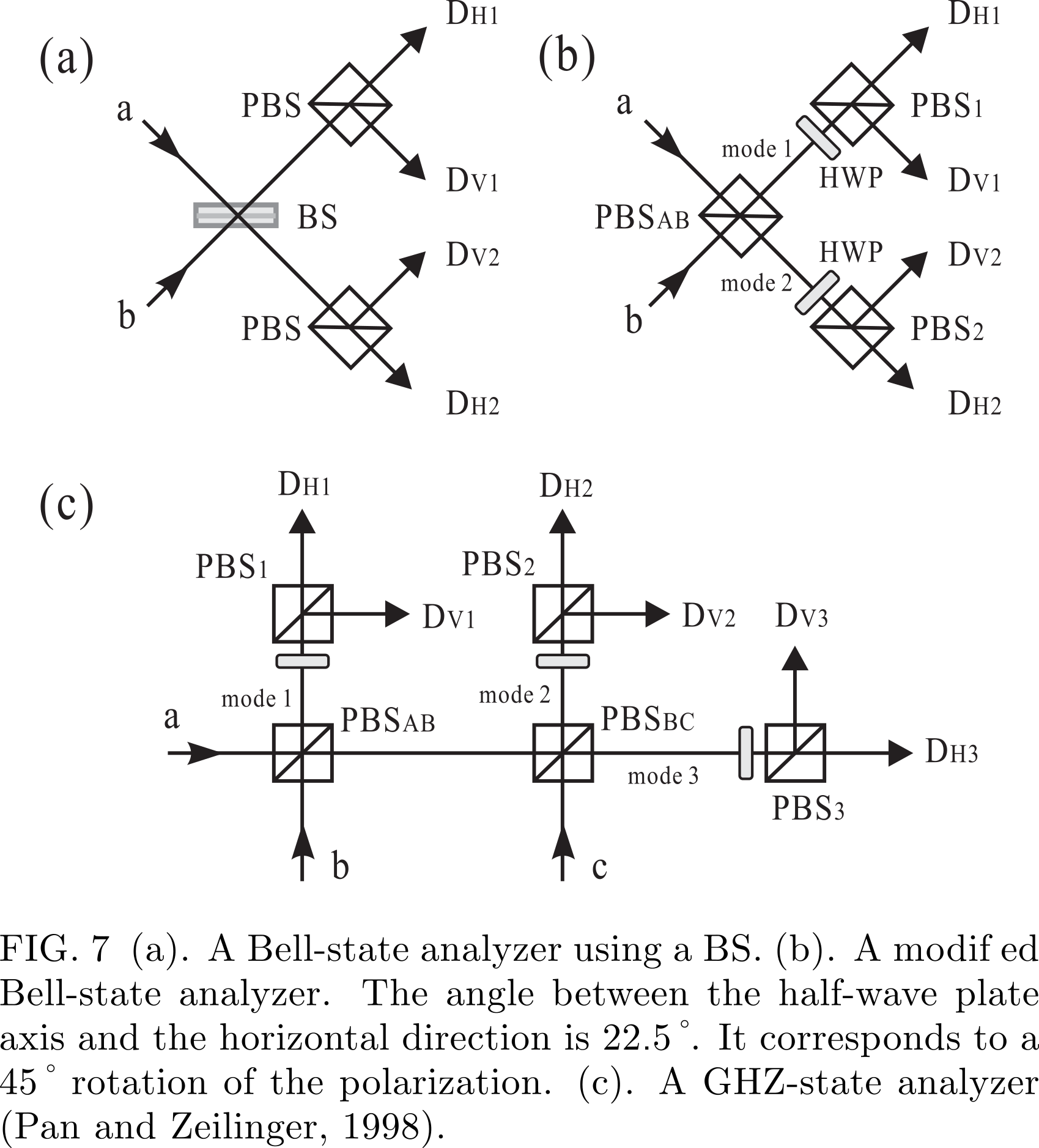#### Bell-state analyzer

Specifically, for the incident state ) we always observe a coincidence either between detectors and or and . On the other hand, if the incident state is ) we observe coincidence at detectors and or and . The other two Bell states would lead to no coincidence at detectors in modes 1 and 2.

### 1.4.2.3.6. GHZ-state analyzer

Bell state measurement schemes can be generalized to the N-particle case. One can construct a GHZ-state analyzer, with which one can identify two out of the maximally entangled GHZ states. In the case of three spectrally indistinguishable identical photons, eight maximally entangled polarization GHZ states could be given by

## 1.4.2.4. EXPERIMENTAL REALIZATIONS OF PHOTONIC ENTANGLEMENT

### 1.4.2.4.1. Spontaneous parametric down-conversion

If one shines strong laser light on a nonlinear crystal, the pump photons have some probability to split into correlated pairs of lower energy. This is called spontaneous parametric down conversion (SPDC). The new photons, customarily called “signal” and “idler”, satisfy the following relations: for the wave vectors within the crystal one has where subscripts 0, s and i denote, respectively, pump, signal and idler wave vectors, and the respective frequencies satisfy . This is usually called phase matching. There are two different types of the process: either signal and idler photons share the same polarization (type I) or they have perpendicular polarizations (type II).

#### Types of entanglement

Polarization entanglement Currently the standard method to produce polarization-entangled photons is the noncollinear type-II SPDC process After the compensation, interference occurs pairwise between processes in which the photon pair is created at distances from the middle of the crystal. The ideal compensation is therefore to use two crystals, one in each path, which are identical with the down-conversion crystal, but only half as long. If the polarizations are rotated by (e.g., with a half wave plate), the retardations of the o and e components are exchanged and temporal indistinguishability is restored. The method also provides reasonable compensation for the transverse walk-off effect. (WHY?)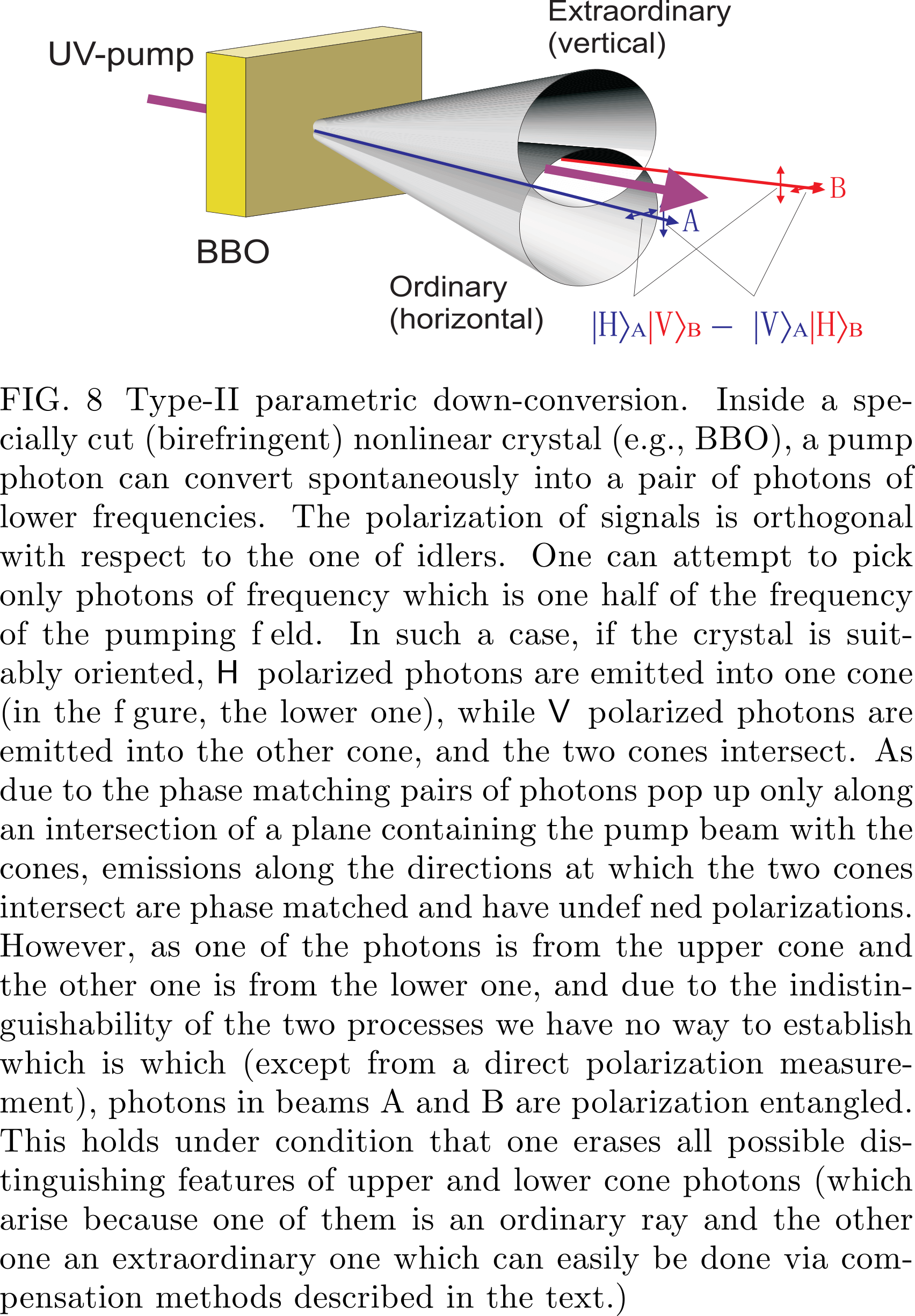Temporal entanglement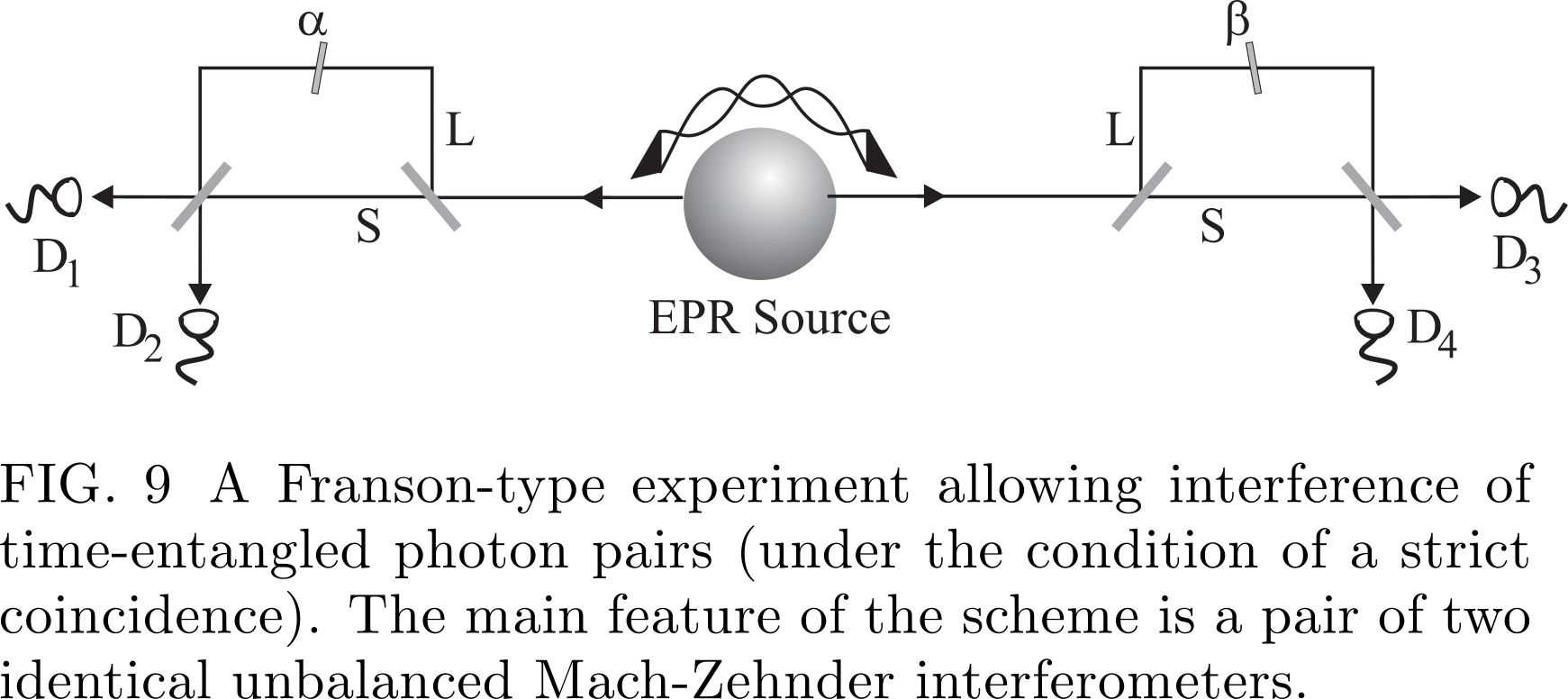An advantage of the time-bin entanglement is that it is insensitive to polarization fluctuations. Using reference laser pulses to actively lock the phase, it can be robustly distributed over long distances in optical fibers.

### 1.4.2.4.2. Photonic entanglement in higher dimensions

#### Entangled qudits

Photonic entanglement in higher dimensions can in principle be generated by SPDC processes in a form of generalization of path or temporal entanglement into more than two conjugate pairs of beams or time-bins, respectively, and analyzed with N-port beamsplitters Another route is to use the photons' orbital angular momentum.

#### Hyper-entanglement

Polarization-path entanglement Polarization-path entanglement can be generated by a double pass of a pump laser through a BBO crystal. The pump passes the crystal and is reflected to pass it again.

Polarization-time entanglement Using a “time-path transmitter” introduced by Chen et al. (2006d), one can realize a transformation between polarization-path and polarization-time hyper-entanglement.

Entanglement in multiple degrees of freedom By pumping two BBO crystals with optical axes aligned in perpendicular planes, a two-photon -dimensional hyperentangled state was produced, approximately described by Here describes the orbital-angular-momentum spatial mode balance which is due the properties of the source and the selection via the mode-matching conditions.

### 1.4.2.4.3. Twin-beam multi-photon entanglement

It is different from multi-photon entanglement in which each spatially separated photon represents a qubit, and can be individually manipulated and observed.

A simplified Hamiltonian responsible for generation of polarization entangled SPDC photons can be put as Horizontally (H) and vertically (V) polarized photons occupy two spatial modes (a and b); is a coupling constant that depends on the nonlinearity of the crystal and the intensity of the pump pulse. After the interaction time t the resulting photon state is given by and in the number state representation reads A special twin-beam entanglement is the so-called “high NOON” type state of two beams: These experiments demonstrated an interesting feature of NOON states: the effective de Broglie wavelength of the multiphoton state is by 1/N shorter than the wavelength of the single photon

### 1.4.2.4.4. Multi-photon entanglement

#### Entanglement construction

Entangling two independent particles: the principle.Note that depending on the phase shifts the detection at, e.g., c(+) and d(+), acts like a Bell-state measurement, projecting the  two photons into the state . The other two photons are, due  to this event, in state: . This process is called entanglement swapping.

Enforcing source indistinguishability.

One could determine that the photon detected at d(+) came from crystal A (B) by noting the near simultaneity of the detection of photon d(+) and one of the photons at a(+) or b(+)

#### New methods

A method gaining great importance is tuning the properties of the downconversion source and the pump such that one obtains frequency uncorrelated pairs of photons

#### First proposals

ssume that sources A and B simultaneously emit a photon pair each. Pairs in beams x, y (1, 3, and 2, 4) are in identical polarization states . The state of the four particles, 2 after the passage of 2 and 3 via PBS1, and provided the photons are indistinguishable (which can be secured using the methods as described earlier), reads Only the superposition , Only the superposition , which is a GHZ state, leads to four-fold coincidence. Therefore, four-fold coincidences can reveal four-particle GHZ correlations. which is a GHZ state, leads to four-fold coincidence. Therefore, four-fold coincidences can reveal four-particle GHZ correlations.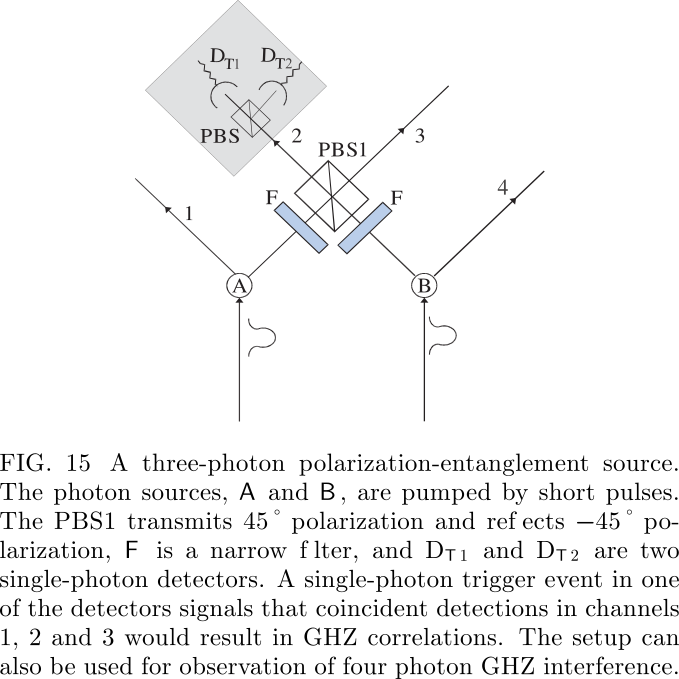Due to the absence of perfect pair sources and perfect single photon detectors, in the experiments both three- and four-photon entanglement is observed only under the condition that there is one and only one photon in each of the four outputs.

## 1.4.2.5. FALSIFICATION OF A REALISTIC WORLD VIEW

Realism, the cornerstone of classical physics, is a view that for any physical system (also a subsystem of a compound system), one can find a theoretical description (deterministic or probabilistic) which involves all results of all possible experiments that can be performed upon it no matter which experiment actually was performed. Evidently, this directly contradicts the Bohr's complementarity principle. A theory is local if it assumes that information, and influences, cannot travel faster than light.

### 1.4.2.5.1. Bell's inequality

The following inequalities must be satisfied for local realistic descriptions

If one chooses a non-factorizable function , say, , the famous CHSH (Clauser, Horne, Shimony, and Holt, 1969) inequality is recovered

## 1.4.2.6. GHZ theorem

### 1.4.2.6.1. Impossibility of deriving realism via perfect quantum correlations and locality

Take the GHZ experiment, Fig. 2. Assume that a click at the local detector , where is described as a result of value +1, whereas clicks at are ascribed −1. According to the quantum probabilities (20) the average values of the product of local results reads

where s the value of a local measurement, by Alice, Bob and Cecil, respectively, that would have been obtained for the n-th particle triple, if the setting of the measuring device is . Now, in a local realistic model, from these results we can deduce a further correlation by simply multiplying Eqs. Since , regardless of whether or −1, we obtain This, however, is in a full contradiction with the quantum mechanical prediction obtained from (*) which reads:

#### Two-observer GHZ-like correlations

Interestingly, the GHZ reasoning can be reduced to a two-party (thus two space-like separated regions) case while its all-versus-nothing feature is still retained. One option is to encode three two-state quantum systems in distinct degrees of freedom of only two photons. The second option is to find suitable EPR elements of reality in the two particle case, and to show that they are internally inconsistent. A third protocol of the two-observer GHZ-like the- orem uses a two-photon hyper-entanglement.

### 1.4.2.6.2. Refutation of a class of nonlocal realistic theories

Let us discuss the description of the polarization of photons within such theories. The following assumptions are made: (L 1 ) realism, (L 2 ) physical states are statistical mixtures of subensembles with definite polarizations, (L 3 ) local expectation values for polarization measurements taken for each subensemble obey Malus' law. Importantly, locality is not assumed.

Leggett-type inequality

where is a uniform average of all correlation functions, defined in the plane of and , with the same relative angle . Inequalities avoiding the averaging were also derived and tested. For the inequality to be applicable, the vectors and necessarily have to lie in a plane orthogonal to the one defined by and . This contrasts with the CHSH inequality.

## 1.4.2.7. QUANTUM COMMUNICATION

Quantum communication ultimately aims at absolute security and faithful transfer of information, classical or quantum.

### 1.4.2.7.1. Quantum dense coding

The idea of quantum dense coding is that, by manipulating only one of the two particles in a Bell state, one can also encode two bits of information.

The procedure runs as follows:

Step 1. Sharing maximal entanglement. A maximally entangled qubit pair (say, in the state ) is shared by Alice and Bob (Fig. 31). They agree in advance that respectively represent the binary numbers 00, 01, 10, and 11.

Step 2. Coding of the message. According to the value Bob wants to transmit to Alice, he performs one out of four possible unitary transformations (identity operation ) on his qubit B alone. The three non- I, identity operations transform, in an one-to-one way, the original state , respectively, into , and . Once this is done, Bob sends his qubit to Alice. Note that this possibility of transforming any of the four basis states to any other by only manipulating one of the two qubits holds only for the maximally entangled states. For product (and classical) states it is always necessary to have control over both qubits (bits) to encode two bits in four distinguishable states.

Step 3. Decoding of the message. Upon reception, Alice performs a Bell-state measurement, distinguishing between the four code-states and thus allowing her to read out both bits of information. The quantum dense coding doubles the information capacity of the transmission channel: what is actually sent is only one qubit. This more efficient way of coding information at first glance seems to be at odds with the Holevo theorem (1973), which states that maximally one bit can be encoded on a single qubit. Entanglement, a property of pairs of qubits, allows to circumvent this theorem and to encode infor- mation entirely in the relative properties of the pair, i.e. in their correlations.

### 1.4.2.7.2. Quantum teleportation

#### Theory: qubit teleportation involving an EPR channel and two bit transfer

see this and this

#### Open-destination quantum teleportation

The so-called open-destination teleportation is a protocol allowing to transfer a state to one of several potential recipients. It can be decided who gets the state even after the initial to-be-teleported state, , is wiped out in a Bell-state measurement.

Figure 24 shows the basic scheme. One uses a quantum channel in form of a N +1 qubit GHZ state, say for N = 3 and for polarization qubits: and requires, as always, a transfer of two bit classical information. The polarization state to-be-teleported is first encoded into an N -particle coherent superposition of these GHZ particles. By making a Bell state measurement on, say, photons 1 and 2, one projects the remaining photons into one of four states. E.g. whenever the result of the Bell-state measurement is a state, one gets The state can be read out at any of the three par- ticles, by performing a suitable projection measurement on N − 1 of them, here on 2, and a unitary transformation dependent of the received two bits of data, which is carried out on one of the GHZ particles. Assume that we want to transfer our state to particle 5. To this end upon the receipt of information concerning the result of the BSM, the partner 5 must perform on his particle a transformation which interchanges polarizations H and V . The partners 3 and 4 make measurements in the basis. The recipient of the state is informed about the measurement results . Once the recipient gets this additional information, only if there was just one result associated with a projection to , he/she performs the sign flipping transformation. The state is recovered.

### 1.4.2.7.3. Entanglement swapping

#### Theory

Entanglement swapping, together with entanglement purification, is a key element of the quantum repeater protocol and opens a way to efficiently distribute entanglement for massive particles. Namely, as soon as Al- ice completes the Bell-state measurement on particles 2 and 3, we know that photons 1 and 4 are on their way, ready for detection in an entangled state. In this way one has the possibility to perform an event-ready test of Bell’s inequality

Halder et al. (2007) took a different approach to achieve entanglement swapping by a precise time measurement. The photon detector used in the experiment was a niobium nitride superconducting single photon detector with a time resolution of 74ps . The photons were filtered using 10pm-bandwidth filters, which corresponds to a coherence time of 350ps well above the temporal resolution of the detectors. Hence, ultra-coincidence photon timing could be obtained, and pulsed sources could be replaced by continuous-wave sources, which do not require any synchronization.

### 1.4.2.7.4. Beating noisy environment

An important tool to overcome the noise in the quan- tum communication channel is entanglement distillation, concentration and purification

#### Entanglement distillation and concentration

Entanglement concentration aims to obtain with a nonzero probability a higher entanglement from pure states with a lower entanglement. There are two methods to achieve this. The first is the so-called Procrustean method. It requires that the photon pairs are all in a pure non-maximally entangled state, say, , where and are two known amplitudes. In this case, the scheme only involves local filtering operations on single pairs. Second, the Schmidt decomposition scheme works for photon pairs that are all in a pure but unknown non-maximally entangled state . In practice, this scheme is more difficult to implement as it requires simultaneous collective measurements on many photons.

#### Entanglement purification

The underlying idea of entanglement purification is that, by using local operations and classical commu- nication (LOCC) only, to extract from multiple copies of imperfect states (arbitrary mixed states) fewer copies of entangled state asymptotically to near-unity fidelity.

The linear-optical purification scheme [shown in Fig. 28(b)] will be presented here using a specific example. Let our initial state be where is an unwanted admixture. The subscripts a and b indicate the particles at Alice's and Bob's locations, respectively.

Alice and Bob share a big number of pairs described by . They start by picking at random two such pairs. Each of them superimposes their photons on a PBS. An essential step in the purification scheme is to select those cases for which there is exactly one photon in each of the four spatial output modes. We shall refer to them as “four-mode cases”. This corresponds to a projection onto the subspace in which two photons at the same experimental location (Alice’s or Bob’s) have equal polarization. This is similar to the bilateral CNOT-operation of the original scheme. Note that the polarizations at two different locations do not have to be the same. After performing the purification procedure (selection of four- mode cases, measurements in modes a4 and b4 in the +/− basis, and local operations conditional on the mea- surement results), Alice and Bob will finally create a new ensemble described by the density operator with a larger fidelity ] (for ) of pairs in the desired state than before thepurification.

Though it seems that only a rather special example, single bit-flip error, has been considered, the same method actually applies to the arbitrary mixed states ρ ab , provided that they contain a sufficiently large fraction F > 1/2 of photon pairs in a maximally entangled state. This works as follows: one can first purify away single bit-flip errors; phase errors can then be easily transformed into bit-flip errors by a polarization rotation and treated in a subsequent purification step.